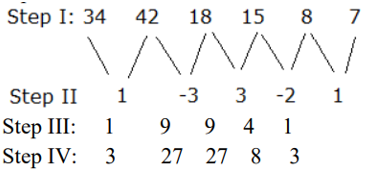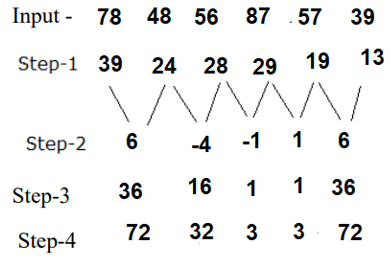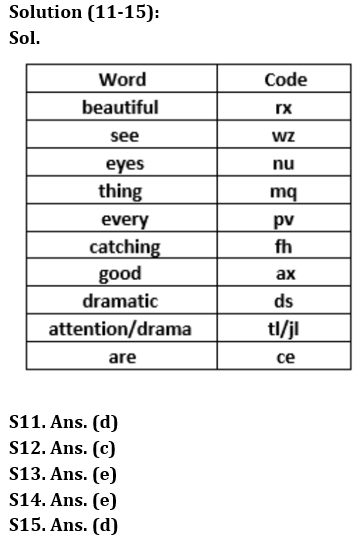Latest Banking jobs   »

# Reasoning Quiz For RBI Grade B Phase 1 2023-16th March

Direction (1-5): A number arrangement machine, when given a particular input of numbers, rearranges them following a particular rule. The following is the illustration of the input and the steps of arrangement.

Input: 21, 38, 18, 20, 56, 72, 48, 44, 76, 82, 60, 32, 28, 94
Step I: 20, 33, 11, 18, 55, 67, 44, 44, 75, 76, 54, 31, 22, 89
Step II: 22, 32, 10, 20, 54, 66, 46, 46, 74, 78, 56, 30, 24, 88
Step III: 9, 14, 3, 8, 25, 31, 21, 21, 35, 37, 26, 13, 10, 42
Step IV: 18, 42, 6, 24, 50, 62, 42, 42, 70, 74, 78, 26, 30, 126
Step V: 9, 36, 6, 18, 45, 54, 36, 36, 63, 63, 63, 18, 27, 117

Step V is the last step of the above arrangement. As per the rules followed in the above steps, find out the answer of each of the following question based on the given input.

32, 25, 62, 34, 83, 65, 73, 92, 36, 41, 89, 46, 30, 52

Q1. Which element is fifth from the right end in step III?
(a) 32
(b) 114
(c) 42
(d) 18
(e) None of these

Q2. Which of the following operation is done in step IV of the given input output?
(a) Numbers are multiplied by 2
(b) Sum of the digits are subtracted from the number
(c) Odd numbers are multiplied by 2 and even numbers by 3
(d) Numbers are divided by sum of their digits
(e) None of these

Q3. Which of the following property is common in the elements present in step V?
(a) All numbers are odd numbers
(b) Each number is divisible by 4
(c) Each number is divisible by 2
(d) Numbers are divisible by 9
(e) Both (b) and (d)

Q4. How many prime numbers are there in step III of the given input?
(a) Three
(b) Four
(c) Seven
(d) Five
(e) Nine

Q5. Which of the following would be step II of the arrangement?
(a) 30, 24, 60, 32, 80, 66, 68, 84, 32, 40, 90, 46, 26, 48
(b) 26, 30, 84, 42, 114, 62, 96, 120, 42, 54, 86, 42, 22, 66
(c) 34, 84, 30, 42, 63, 119, 96, 120, 42, 54, 86, 42, 22, 66
(d) 31, 22, 58, 33, 71, 64, 69, 85, 35, 38, 88, 44, 27, 49
(e) 35, 22, 58, 33, 74, 64, 69, 85, 33, 33, 88, 44, 27, 49

Directions (6-10): A number arrangement machine when given an input line of numbers rearranges them following a particular rule in each step. The following is an illustration of input and rearrangement.

Input: 68 84 36 45 16 21Step V is the last step of the above arrangement. As per rules followed in the above steps, find out in each of the given questions the appropriate steps for the given input.

Input – 78 48 56 87 57 39

Q6. What will be the sum of numbers present in step II?
(a) 13
(b) 14
(c) 8
(d) 11
(e) None of the above

Q7. Which element is 4th from the right end in step IV of the given input?
(a) 32
(b) 76
(c) 72
(d) 3
(e) None of these

Q8. Which among the following is the last element (from the left end) in step III of the given input?
(a) 16
(b) 36
(c) 18
(d) 1
(e) None of these

Q9. Which of the following would be the Step I?
(a) 39 24 28 29 19 13
(b) 24 39 28 29 13 19
(c) 39 28 24 29 19 13
(d) 24 28 39 19 13 24
(e) None of these

Q10. What will be the sum of numbers present in step IV?
(a) 184
(b) 176
(c) 182
(d) 181
(e) None of these

Direction (11-15): Study the following information carefully and answer the given questions.
In c certain code language
‘Eyes see beautiful thing’ is written as ‘wz mq nu rx’,
‘Beautiful thing are catching’ is written as ‘rx ce fh mq’,
‘Every attention eyes drama’ is written as ‘nu tl pv jl’,
‘Dramatic catching every eyes’ is written as ‘fh nu ds pv’,
‘Every good see beautiful’ is written as ‘ax rx pv wz’

Q11. What is the code for ‘every’ and ‘beautiful’?
(a) rx and ax
(b) wz and pv
(c) mq and ce
(d) pv and rx
(e) nu and mq

Q12. If ‘See eyes drama catching’ is coded as ‘tl wz nu fh’ then what will be the possible code for ‘thing are attention’?
(a) wz ds pv
(b) ce rx jl
(c) mq jl ce
(d) ax mq ds
(e) Cannot be determined

Q13. What is the word for the code ‘wz’?
(a) Good
(b) Eyes
(c) Thing
(d) Beautiful
(e) See

Q14. What are the words coded as ‘pv gt ds jl ce’?
(a) Dramatic drama are every kind
(b) Attention wonders dramatic are drama
(c) every attention wonderful are dramatic
(d) None of these
(e) Either (a) or (c)

Q15. If the code of ‘see’ is interchanged with the code of ‘eyes’ then what will be the code for ‘beautiful eyes’ and ‘see thing’ respectively?
(a) ‘fh ds’ and ‘nu ce’
(b) ‘ax nu’ and ‘ce rx’
(c) ‘wz mq’ and ‘ax fh’
(d) ‘rx wz’ and ‘nu mq
(e) Can’t be determined

Solutions

Solutions(1-5):
Sol.

Logic: Step I: Difference between the digits of the number is subtracted from the number.
Step II: Add 2 to even numbers and subtract 1 from odd numbers.
Step III: Divide each number by 2 and then subtract 2 from it.
Step IV: multiply odd numbers by 2 and even numbers by 3.
Step V: Subtract the sum of the digits from the number.

Input: 32, 25, 62, 34, 83, 65, 73, 92, 36, 41, 89, 46, 30, 52
Step I: 31, 22, 58, 33, 78, 64, 69, 85, 33, 38, 88, 44, 27, 49
Step II: 30, 24, 60, 32, 80, 66, 68, 84, 32, 40, 90, 46, 26, 48
Step III: 13, 10, 28, 14, 38, 31, 32, 40, 14, 18, 43, 21, 11, 22
Step IV: 26, 30, 84, 42, 114, 62, 96, 120, 42, 54, 86, 42, 22, 66
Step V: 18, 27, 72, 36, 108, 54, 81, 117, 36, 45, 72, 36, 18, 54

S1. Ans.(d)
S2. Ans.(c)
S3. Ans.(d)
S4. Ans.(b)
S5. Ans.(a)

Solution (6-10):
Sol.
Step 1 Even number is divided by 2 and odd number is divided by 3
Step-2 Addition of 1st digit and 2nd digit of 2nd number and is subtracted from Addition of 1st and 2nd digit of 1st number. Then, Addition of 1st digit and 2nd digit of 3rd number and is subtracted from Addition of 1st and 2nd digit of 2nd number and so on.
Step-3 Squaring of all the numbers
Step-4 Even numbers are multiplied by 2 and odd numbers are multiplied by 3S6. Ans.(c)
S7. Ans.(a)
S8. Ans.(b)
S9. Ans.(a)
S10. Ans.(c)## FAQs

### What is the selection process of the Bank Clerk?

There are 4 sections in the RBI Grade B Phase 1 Exam i.e. English Language, General Awareness, Quantitative Aptitude & Reasoning.

#### Congratulations!Union Budget 2023-24: Free PDF